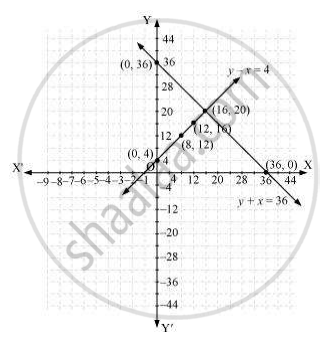# Half the perimeter of a rectangular garden, whose length is 4 m more than its width, is 36 m. Find the dimensions of the garden - Mathematics

Half the perimeter of a rectangular garden, whose length is 4 m more than its width, is 36 m. Find the dimensions of the garden

#### Solution

Let the width of the garden be x and length be y.

According to the question

y - x = 4 (1)

y + x = 36 (2)

y - x = 4

y = x + 4

 x 0 8 12 y 4 12 16

y + x = 36

 x 0 36 16 y 36 0 20

Hence, the graphic representation is as followsFrom the figure, it can be observed that these lines are intersecting each other at only point i.e., (16, 20). Therefore, the length and width of the given garden is 20 m and 16  respectively.

Concept: Graphical Method of Solution of a Pair of Linear Equations
Is there an error in this question or solution?

#### APPEARS IN

NCERT Class 10 Maths
Chapter 3 Pair of Linear Equations in Two Variables
Exercise 3.2 | Q 5 | Page 50